Courses

# Synchronous Generator Operation Notes | EduRev

## Electrical Engineering (EE) : Synchronous Generator Operation Notes | EduRev

The document Synchronous Generator Operation Notes | EduRev is a part of the Electrical Engineering (EE) Course Electrical Machines for Electrical Engg..
All you need of Electrical Engineering (EE) at this link: Electrical Engineering (EE)

Cylindrical Rotor Machine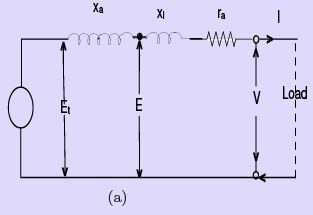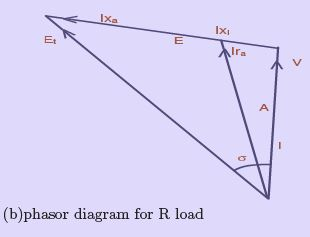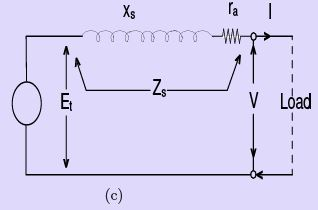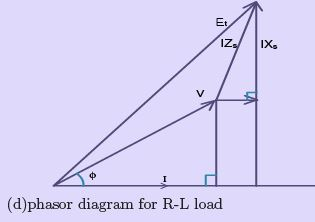Figure 30: Equivalent circuits

The synchronous generator, under the assumption of constant synchronous reactance, may be considered as representable by an equivalent circuit comprising an ideal winding in which an e.m.f. Eproportional to the ﬁeld excitation is developed, the winding being connected to the terminals of the machine through a resistance ra and reactance (Xl + Xa ) = Xs all per phase. This is shown in Fig. 30. The principal characteristics of the synchronous generator will be obtained qualitatively from this circuit.

Consider a synchronous generator driven at constant speed and with constant excitation. On open circuit the terminal voltage V is the same as the open circuit e.m.f. Et .
Suppose a unity-power-factor load be connected to the machine. The ﬂow of load current produces a voltage drop I Zs in the synchronous impedance, and terminal voltage V is reduced. Fig. 31 shows the complexor diagram for three types of load. It will be seen that the angle σ between Et and V increases with load, indicating a shift of the ﬂux across the pole faces due to cross- magnetization. The terminal voltage is obtained from the complex summation

V + Zs = Et or V = Et − I Zs                                        (24)

Algebraically this can be written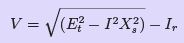(25)

for non-reactive loads. Since normally r is small compared with Xs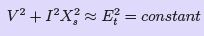(26)
so that the V/I curve, Fig. 32, is nearly an ellipse with semi-axes Et and Isc. The current Isc is that which ﬂows when the load resistance is reduced to zero. The voltage V falls to zero also and the machine is on short-circuit with V = 0 and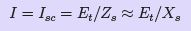(27)

For a lagging load of zero power-factor, diagram is given in Fig. 31 The voltage is given as before and since the resistance in normal machines is small compared with the synchronous reactance, the voltage is given approximately by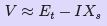(28)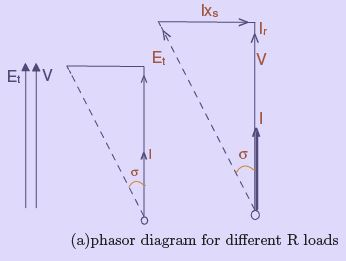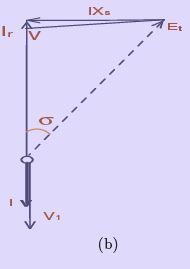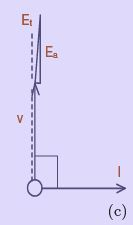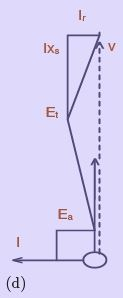Figure 31: Variation of voltage with load at constant Excitation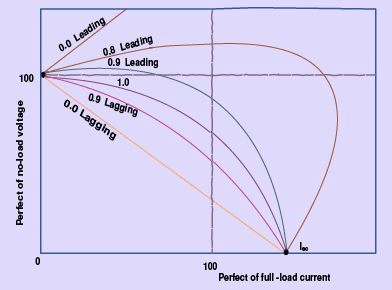which is the straight line marked for cos φ = 0 lagging in Fig. 32. A leading load of zero power factor Fig. 31. will have the voltage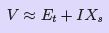(29)

another straight line for which, by reason of the direct magnetizing eﬀect of leading currents, the voltage increases with load.

Intermediate load power factors produce voltage/current characteristics resembling those in Fig. 32. The voltage-drop with load (i.e. the regulation) is clearly dependent upon the power factor of the load. The short-circuit current Isc at which the load terminal voltage falls to zero may be about 150 per cent (1.5 per unit) of normal current in large modern machines.

Generator Voltage-Regulation

The voltage-regulation of a synchronous generator is the voltage rise at the terminals when a given load is thrown oﬀ, the excitation and speed remaining constant. The voltagerise is clearly the numerical diﬀerence between Et and V, where V is the terminal voltage for a given load and Eis the open-circuit voltage for the same ﬁeld excitation. Expressed

as a fraction, the regulation is

ε = (Et − V )/V perunit                                     (30)

Comparing the voltages on full load (1.0 per unit normal current) in Fig. 32, it will be seen that much depends on the power factor of the load. For unity and lagging power factors there is always a voltage drop with increase of load, but for a certain leading power factor the full-load regulation is zero, i.e. the terminal voltage is the same for both full and no-load conditions. At lower leading power factors the voltage rises with increase of load, and the regulation is negative. From Fig. 30, the regulation for a load current I at power factor cos φ is obtained from the equality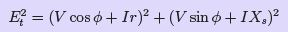(31)

from which the regulation is calculated, when both Et and V are known or found.

Generator excitation for constant voltage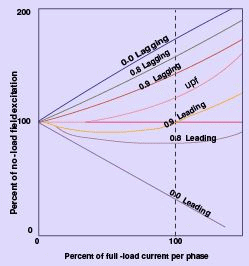Figure 33: Generator Excitation for constant Voltage

Since the e.m.f. Eis proportional to the excitation when the synchronous reactance is constant, the Eqn. 31 can be applied directly to obtain the excitation necessary to maintain constant output voltage for all loads. All unity-and lagging power-factor loads will require an increase of excitation with increase of load current, as a corollary of Fig. 32.

Low-leading-power-factor loads, on the other hand, will require the excitation to be reduced on account of the direct magnetizing eﬀect of the zero- power-factor component. Fig. 33 shows typical e.m.f./current curves for a constant output voltage. The ordinates of Fig. 33 are marked in percentage of no-load ﬁeld excitation, to which the e.m.f Et exactly corresponds when saturation is neglected.

Generator input and output

For any load conditions as represented by Fig. 30, the output per phase is P = V I cos φ. The electrical power converted from mechanical power input is per phase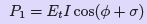(32)
Resolving Et along I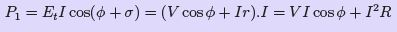(33)

The electrical input is thus the output plus the I 2 R loss, as might be expected. The prime mover must naturally supply also the friction, windage and core losses, which do not appear in the phasor diagram.
In large machines the resistance is small compared with the synchronous reactance so that θ = arc tan(xs/r) ≈ 90, it can be shown that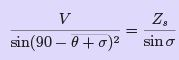(34)
and hence,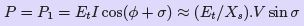(35)

Thus the power developed by a synchronous machine with given values of EV and Zs is proportional to sinσ: or, for small angles, to σ, and the displacement angle σ representing the change in relative position between the rotor and resultant pole- axes is proportional to the load power. The term load-, power- or torque-angle may be applied to σ.
An obvious deduction from the above Eqn. 35is that the greater the ﬁeld excitation (corresponding to E) the greater is the output per unit angle σ: that is, the more stable will be the operation.

Salient Pole Rotor Machine

As discussed earlier in Sec. 3.1 the behaviour of a synchronous machine on load can be determined by the use of synchronous reactance xs which is nothing but the sum of xa and x, where xa is a ﬁctitious reactance representing the eﬀect of armature reaction while xl is the leakage reactance. It was also mentioned that this method of representing the eﬀect of armature reaction by a ﬁctitious reactance xa was applicable more aptly only for a cylindrical rotor (non-salient pole) machine. This was so as the procedure followed therein was valid only when both the armature and main ﬁeld m.m.f.’s act upon the same magnetic circuit and saturation eﬀects are absent.

Theory of Salient-pole machines (Blondel’s Two-reaction Theory)

It was shown in Sec. ?? that the eﬀect of armature reaction in the case of a salient pole synchronous machine can be taken as two components - one acting along the direct axis (coinciding with the main ﬁeld pole axis) and the other acting along the quadrature axis (inter-polar region or magnetic neutral axis) - and as such the mmf components of armature-reaction in a salient-pole machine cannot be considered as acting on the same magnetic circuit. Hence the eﬀect of the armature reaction cannot be taken into account by considering only the synchronous reactance, in the case of a salient pole synchronous machine.

In fact, the direct-axis component Fad acts over a magnetic circuit identical with that of the main ﬁeld system and produces a comparable eﬀect while the quadrature-axis component Faq acts along the interpolar space, resulting in an altogether smaller eﬀect and, in addition, a ﬂux distribution totally diﬀerent from that of Fad or the main ﬁeld m.m.f.

This explains why the application of cylindrical-rotor theory to salient-pole machines for predicting the performance gives results not conforming to the performance obtained from an actual test.

Blondel’s two-reaction theory considers the eﬀects of the quadrature and direct-axis components of the armature reaction separately. Neglecting saturation, their diﬀerent eﬀects are considered by assigning to each an appropriate value of armature-reaction “reactance,” respectively xad and xaq . The eﬀects of armature resistance and true leakage reactance ( xl ) may be treated separately, or may be added to the armature reaction coeﬃcients on the assumption that they are the same, for either the direct-axis or quadrature-axis components of the armature current (which is almost true). Thus the combined reactance values can be expressed as :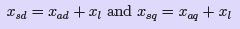(36)

for the direct- and cross-reaction axes respectively. These values can be determined experimentally as described in Sec. 3.2.3

In a salient-pole machine, xaq , the cross- or quadrature-axis reactance is smaller than xad , the direct-axis reactance, since the ﬂux produced by a given current component in that axis is smaller as the reluctance of the magnetic path consists mostly of the interpolar spaces.

It is essential to clearly note the diﬀerence between the quadrature- and direct-axis components Iaq , and Iad of the armature current Ia, and the reactive and active components Iaa and Iar . Although both pairs are represented by phasors in phase quadrature, the former are related to the induced emf Et while the latter are referred to the terminal voltage V .

These phasors are clearly indicated with reference to the phasor diagram of a (salient pole) synchronous generator supplying a lagging power factor (pf ) load, shown in Fig. ??(a). We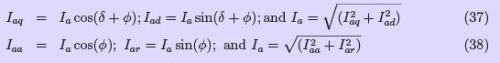where σ = torque or power angle and φ = the p.f. angle of the load.
The phasor diagram Fig. 34 shows the two reactance voltage components Iaq ∗ xsq and Iad ∗ xsd which are in quadrature with their respective components of the armature current.
The resistance drop I∗ Ra is added in phase with Ia although we could take it as Iaq ∗ Ra and Iad ∗ Ra separately, which is unnecessary as

Ia = Iad + j Iaq

Actually it is not possible to straight-away draw this phasor diagram as the power angle σ is unknown until the two reactance voltage components Iaq ∗ xsq and Iad ∗ xsd are known. However this diﬃculty can be easily overcome by following the simple geometrical construction shown in Fig. 34(d), assuming that the values for terminal voltage V , the load power factor (pf ) angle φ and the two synchronous reactances xsd and xsq are known to us.
The resistance drop Ia ∗ Ra (length AB) is added to the tip of the voltage phasor (OA) in phase with the current phasor (i.e. in a direction parallel to OQ ). Then we draw

line BC ( of length equal to Ia ∗ xsq ) and extend it to D such that BD will be (of length equal to Ia ∗ xsd ) at the extremity B of I∗ Ra and at right-angles to Ia . Draw OC and extend it (to F ). From D draw the perpendicular DF on OC extended. Then OF represents the induced voltage Et. The proof for this can be given as follows:. If DF is extended to G such that this line is perpendicular to BG drawn parallel to OF, we have :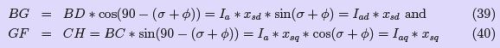Power relations in a Salient Pole Synchronous Machine:

Neglecting the armature winding resistance, the power output of the generator is given by:
P = V ∗ Ia ∗ cos φ                      (41)

This can be expressed in terms of σ, by noting from Fig. 34 that :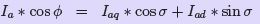(42)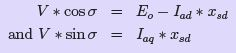Substituting these in the expression for power, we have.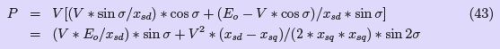It is clear from the above expression that the power is a little more than that for a cylindrical rotor synchronous machine, as the ﬁrst term alone represents the power for a cylindrical rotor synchronous machine. A term in (sin 2σ) is added into the power - angle characteristic of a non-salient pole synchronous machine. This also shows that it is possible to generate an emf even if the excitation Eis zero. However this magnitude is quite less compared with that obtained with a ﬁnite E0. Likewise we can show that the machine develops a torque - called the reluctance torque - as this torque is developed due to the variation of the reluctance in the magnetic circuit even if the excitation E0 is zero.

Experimental Determination of xd and xq

The unsaturated values of xd and xq of a 3-Phase synchronous machine can be easily determined experimentally by conducting the following test known as slip test. The rotor of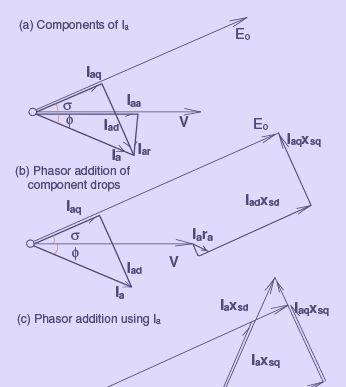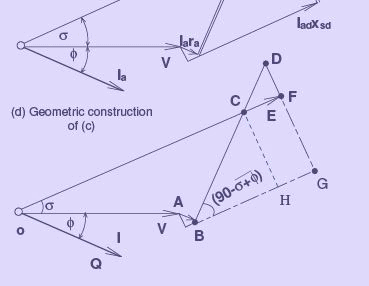Figure 34: Phasor diagram of a generator-Two reaction theory

the synchronous machine is driven by means of a prime mover (usually a DC motor in the laboratory) at a speed close to the synchronous speed in the proper direction but not equal to it. The armature is supplied with a low voltage 3-Phase balanced supply through a variac, while the ﬁeld circuit is kept open. The armature current varies between two limits since it moves through, since the synchronously rotating armature mmf acts through the varying magnetic reluctance paths as it goes from inter-polar axis to pole axis region. The values of xsd and xsq are determined based on the applied voltage and the armature current values.
The ratio of applied voltage to the minimum value of the armature current gives the direct axis synchronous reactance xsd which is usually the same as the synchronous reactance xs that we usually determine from normal no-load and short-circuit tests as explained in Sec. ?? The ratio of applied voltage to the maximum value of the armature current gives the the quadrature-axis reactance xsq . For more accurate determination of these values the oscillogram of the armature current and voltage can be recorded.

Losses and Eﬃciency

To calculate the eﬃciency of a synchronous generator, a procedure is to be followed for establishing the total losses when operating under load. For generators these losses are,

1. Rotational losses such as friction and windage.

2. Eddy current and hysteresis losses in the magnetic circuit

3. Copper losses in the armature winding and in the ﬁeld coils

4. Load loss due to armature leakage ﬂux causing eddy current and hysteresis losses in the armature-surrounding iron.

1. The rotational losses, which include friction and windage losses, are constant, since the speed of a synchronous generator is constant. It may be determined from a no-load test.
2. The core loss includes eddy current and hysteresis losses as a result of normal ﬂux density changes. It can be determined by measuring the power input to an auxiliary motor used to drive the generator at no load, with and without the ﬁeld excited. The diﬀerence in power measured constitutes this loss.

3. The armature and ﬁeld copper losses are obtained as Ia2Rand Vf If Since per phase quantities are dealt with, the armature copper loss for the generator must be multiplied by the number of phases. The ﬁeld winding loss is as a result of the excitation current ﬂowing through the resistance of the ﬁeld winding.

4. Load loss or stray losses result from eddy currents in the armature conductors and increased core losses due to distorted magnetic ﬁelds. Although it is possible to separate this loss by tests, in calculating the eﬃciency, it may be accounted for by taking the eﬀective armature resistance rather than the dc resistance.
After all the foregoing losses have been determined, the eﬃciency η is calculated as,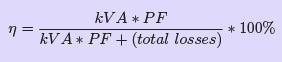(44)
where η = eﬃciency,
kvA = load on the generator (output) PF = power factor of the load
The quantity (kVA*PF) is, of course, the real power delivered to the load (in kW) by the synchronous generator. Thus, it could in general be stated as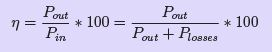(45)
The input power Pin = Pout + Plosses is the power required from the prime mover to drive the loaded generator.

Offer running on EduRev: Apply code STAYHOME200 to get INR 200 off on our premium plan EduRev Infinity!

## Electrical Machines for Electrical Engg.

22 videos|41 docs|26 tests

,

,

,

,

,

,

,

,

,

,

,

,

,

,

,

,

,

,

,

,

,

;